JMSLTM Numerical Library 7.2.0
com.imsl.math

## Class Spline2DInterpolate

• All Implemented Interfaces:
Serializable, Cloneable

```public class Spline2DInterpolate
extends Spline2D```
Computes a two-dimensional, tensor-product spline interpolant from two-dimensional, tensor-product data.

The class `Spline2DInterpolate` computes a tensor-product spline interpolant. The tensor-product spline interpolant to data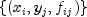, where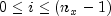and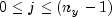has the form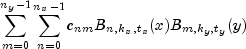where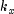and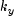are the orders of the splines. These numbers are defaulted to be 4, but can be set to any positive integer using `xOrder` and `yOrder` in the constructor. Likewise,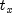and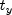are the corresponding knot sequences (`xKnots` and `yKnots`). These default values are selected by `Spline2DInterpolate`. The algorithm requires that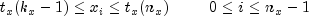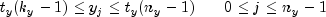Tensor-product spline interpolants in two dimensions can be computed quite efficiently by solving (repeatedly) two univariate interpolation problems.

The computation is motivated by the following observations. It is necessary to solve the system of equations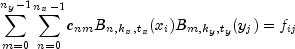Setting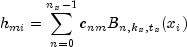note that for each fixed i from 0 to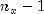, we have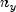linear equations in the same number of unknowns as can be seen below: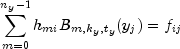Setting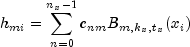note that for each fixed i from 0 to, we have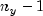linear equations in the same number of unknowns as can be seen below: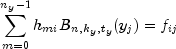The same matrix appears in all of the equations above: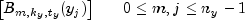Thus, only factor this matrix once and then apply this factorization to the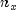right-hand sides. Once this is done and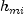is computed, then solve for the coefficients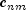using the relation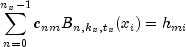for n from 0 to, which again involves one factorization andsolutions to the different right-hand sides. The class `Spline2DInterpolate` is based on the routine SPLI2D by de Boor (1978, p. 347).

Example 1, Example 2, Example 3, Example 4, Serialized Form
• ### Constructor Summary

Constructors
Constructor and Description
```Spline2DInterpolate(double[] xData, double[] yData, double[][] fData)```
Constructor for `Spline2DInterpolate`.
```Spline2DInterpolate(double[] xData, double[] yData, double[][] fData, int xOrder, int yOrder)```
Constructor for `Spline2DInterpolate`.
```Spline2DInterpolate(double[] xData, double[] yData, double[][] fData, int xOrder, int yOrder, double[] xKnots, double[] yKnots)```
Constructor for `Spline2DInterpolate`.

• ### Methods inherited from class com.imsl.math.Spline2D

`derivative, derivative, getCoefficients, getXKnots, getYKnots, integral, value, value`
• ### Methods inherited from class java.lang.Object

`clone, equals, finalize, getClass, hashCode, notify, notifyAll, toString, wait, wait, wait`
• ### Constructor Detail

• #### Spline2DInterpolate

```public Spline2DInterpolate(double[] xData,
double[] yData,
double[][] fData)```
Constructor for `Spline2DInterpolate`.
Parameters:
`xData` - a `double` array containing the data points in the x-direction.
`yData` - a `double` array containing the data points in the y-direction.
`fData` - a `double` matrix of size `xData.length` by `yData.length` containing the values to be interpolated.
• #### Spline2DInterpolate

```public Spline2DInterpolate(double[] xData,
double[] yData,
double[][] fData,
int xOrder,
int yOrder)```
Constructor for `Spline2DInterpolate`.
Parameters:
`xData` - a `double` array containing the data points in the x-direction.
`yData` - a `double` array containing the data points in the y-direction.
`fData` - a `double` matrix of size `xData.length` by `yData.length` containing the values to be interpolated.
`xOrder` - an `int` scalar value specifying the order of the spline in the x-direction. `xOrder` must be at least 1. Default: `xOrder` = 4, tensor-product cubic spline.
`yOrder` - an `int` scalar value specifying the order of the spline in the y-direction. `yOrder` must be at least 1. Default: `yOrder` = 4, tensor-product cubic spline.
• #### Spline2DInterpolate

```public Spline2DInterpolate(double[] xData,
double[] yData,
double[][] fData,
int xOrder,
int yOrder,
double[] xKnots,
double[] yKnots)```
Constructor for `Spline2DInterpolate`.
Parameters:
`xData` - a `double` array containing the data points in the x-direction.
`yData` - a `double` array containing the data points in the y-direction.
`fData` - a `double` matrix of size `xData.length` by `yData.length` containing the values to be interpolated.
`xOrder` - an `int` scalar value specifying the order of the spline in the x-direction. `xOrder` must be at least 1. Default: `xOrder` = 4, tensor-product cubic spline.
`yOrder` - an `int` scalar value specifying the order of the spline in the y-direction. `yOrder` must be at least 1. Default: `yOrder` = 4, tensor-product cubic spline.
`xKnots` - a `double` array of size `xData.length + xOrder` specifying the knot sequences of the spline in the x-direction. Default knot sequences are selected by the class.
`yKnots` - a `double` array of size `yData.length + yOrder` specifying the knot sequences of the spline in the y-direction. Default knot sequences are selected by the class.
JMSLTM Numerical Library 7.2.0